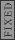GR 8677927796770177 | # Login | Register

GR9277 #30
Problem
 GREPhysics.NET Official Solution Alternate Solutions
\prob{30}
Given that the binding energy of the hydrogen atom ground state is 13.6eV, the binding energy of the n=2 state of positronium (positron-electron system) is

Quantum Mechanics}Bohr Theory

The ground state binding energy of positronium is half of that of Hydrogen. This is so because the energy is proportional to the reduced mass, and that of the positronium has a reduced mass of half that of Hydrogen.

Thus, from the Bohr formula, one has , where and is the ground state energy of Hydrogen. (The ground state energy of positronium is half that of Hydrogen because its reduced mass is half that of Hydrogen's.)

Since , then for , the energy is , as in choice (E).Alternate Solutions
 There are no Alternate Solutions for this problem. Be the first to post one!
bucky0
2007-10-04 17:11:46
For positronium, I always assume that 6.8 eV is the binding energy. It's quick and right.agaliarept
2006-11-25 16:00:09
How can Z=1 when there is no Proton or Neutoron in positronium?
 Jeremy2007-10-30 18:11:20 In this formula, is used to represent the number of positive charges in the nucleus. In typical cases, this represents the number of protons. Here however, it is a positron that contributes the charge.tera
2006-08-21 04:58:39
Positron has 1/1800 the mass of hydrogen i think and i can not see the formula tha says that energey is proportional to the mass. I just can not understand the correct answer

 caffeinated2008-04-09 10:13:33 The Rydberg constant is E/hc =911.27E-1 angstoms. For H, divide by 1+m/M which is the reduced mass. In this case, M=m, since the nucleus is a positron, it has the same mass as the electron. Thus, the ratio of energy is 1/2.
 dcan2008-04-10 10:00:49 Oops. I left out . The Rydberg constant is . I sometimes forget about Z because it's usually H with Z=1.
 Almno102010-11-12 14:02:03 The reduced mass of hydrogen atom is about me, since mu = (mp*me)/(mp + me). Do this for positronium, and see that the reduced mass is (1/2)me
 Marian2016-01-05 08:59:41 Neige Hunter: montres, nerds pourrait knock réplique Breitling Bentley Flying B plongée en apnée, il est prêt, durée du rythme cardiaque, mais tout le monde peut être dit de se concentrer sur la conception, \"et alors, regardez cette heure génial !.\"\r\n\r\nhttp://www.repliquemontresfr.euastro_allison
2005-11-24 04:08:02
are you sure z=2 here? the math doesn't work. plus it doesn't make sense that positronium, with a smaller reduced mass than helium (z=1) would have a higher z. shouldn't z=-1?
 yosun2005-11-26 01:34:59 astro_allison: thanks for the typo-alert; it has been corrected.LaTeX syntax supported through dollar sign wrappers $, ex.,$\alpha^2_0$produces . type this... to get...$\int_0^\infty\partial\Rightarrow\ddot{x},\dot{x}\sqrt{z}\langle my \rangle\left( abacadabra \right)_{me}\vec{E}\frac{a}{b}\$